A view into the mind of Jason

Welcome to Evilness
Monday, January 17 2022 @ 06:32 MST

F stops between f numbers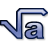For the number of f stops between two f numbers (e.g. f11.8 and 7.4).

Miles Per Gallon to Litres per 100 kmFor MPG given in US gallons, use this formula: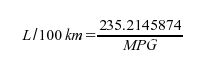For MPG given in Imperial gallons, use this formula: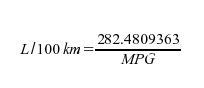Both yield L/100km.

Voltage Divider FormulaFor a given voltage divider: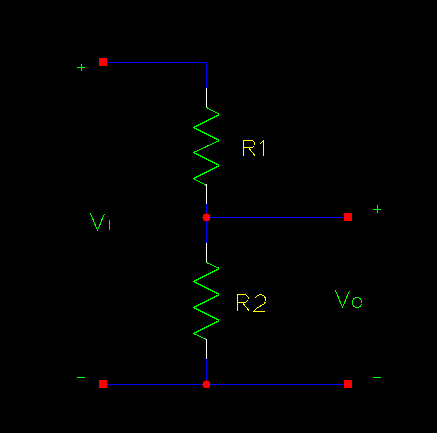To work out the output voltage Vo: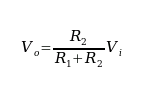To work out the value of R1 for a particular Vi and Vo:Where R1 and R2 are resistances in ohms and Vi and Vo are potentials in volts.

Formula SectionThe purpose of this section is to store formulas that I have found useful and don't want to have to remember or hunt down again.